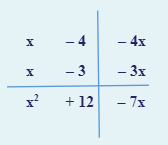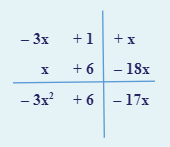Study S2 Mathematics Maths Quadratic Equations and Graphs - GeniebookIn this chapter, we will be discussing the below-mentioned topics in detail:

• Solving quadratic equations in one variable by factorisation

Let’s understand the concept with the help of an example:

\begin{align} 3 \times 0 &= 0 \\ 0 \times (-7) &= 0\\ p \times q &= pq \end{align}

If $$p$$ times $$q$$ is equal to $$0$$, in that case, either $$p$$ is equal to $$0$$ or $$q$$ is equal to $$0$$.

The product of $$p$$ and $$q$$ is zero, but it is not certain whether $$p$$ or $$q$$ is zero, so because of that, we have used the word "or", i.e. either $$p$$ is $$0$$ "or" $$q$$ is $$0$$.

## Zero Product Principle

If we have two unknowns multiplied together and the products of two unknowns are zero, that means either one of the unknowns is zero.

If $$A$$ and $$B$$ are algebraic expressions such that $$AB = 0$$, then $$A=0$$ or $$B = 0$$.

Let’s understand this with the help of some examples:

Question 1:

1.  $$x (x + 3) = 0$$
2.  $$x (3x – 6) = 0$$

Solution:

1. $$x (x + 3) = 0$$

\begin{align} \text{Either} \; x=0 \quad \text{or} \quad x+3 &=0 \\ \\ x &=-3 \end{align}

Linear equations give us one value of $$x$$, but in quadratic equations, generally and not always, we will get two values of $$x$$.

1. $$x (3x – 6) = 0$$

\begin{align} \text{Either} \; x=0 \quad \text{or} \quad 3x-6 &=0 \\ \\ 3x &=6 \\ \\ x &=2 \end{align}

Question 2:

1.  \begin{align} x^2 – 7x &= 0 \end{align}
2.  \begin{align} 6x^2 + 21x = 0 \end{align}

Solution:

1.     \begin{align} x^2 – 7x &= 0 \end{align}

\begin{align} x (x-7) &= 0 \end{align} ​

\begin{align} \text{Either}\; x = 0, \quad \text{or} \quad x – 7 &= 0 \\ \\ x &= 7 \end{align}

1.  \begin{align} 6x^2 + 21x &= 0 \end{align}

\begin{align} 3x (2x + 7) &= 0 \end{align}

\begin{align} \text{Either}\; 3x &= 0,& \text{or} \qquad 2x + 7 &= 0 \\ \\ x &= 0 & 2x &= -7 \\ \\ && x &= -3\frac {1}{2} \end{align}

Question 3:

1. \begin{align} x^2 + 6x + 9 = 0 \end{align}
2. \begin{align} x^2 – 10x +25 = 0 \end{align}
3. \begin{align} 4x^2 – 9 &= 0 \end{align}

Solution:

1. \begin{align} x^2 + 6x + 9 = 0 \end{align}

We know that, \begin{align} (a + b)^2 &= a^2 + 2ab + b^2 \end{align}

\begin{align} (x)^2 + 2 (x)(3) + (3)^2 &= 0 \\ \\ (x + 3)^2 &= 0 \\ \\ x + 3 &= 0 \\ \\ x &= -3 \end{align}

1. \begin{align} x^2 – 10x +25 = 0 \end{align}

We know that, \begin{align} (a - b)^2 &= a^2 - 2ab + b^2 \end{align}

\begin{align} (x)^2 – 2(x)(5) + (5)^2 &= 0 \\ \\ (x – 5)^2 &= 0 \\ \\ x – 5 &= 0 \\ \\ x &= 5 \end{align}

1. \begin{align} 4x^2 – 9 &= 0 \end{align}

We know that,  \begin{align} a^2 – b^2 &= (a+b)(a-b) \end{align}

\begin{align} (2x)^2 – (3)^2 &= 0 \\ \\ (2x + 3) (2x - 3) &= 0 \\\\ \text{Either}\qquad 2x + 3 &= 0 &\text{or} && 2x – 3 &= 0 \\ \\ 2x &= -3 &\text{or} && 2x &= 3 \\ \\ x &= -1.5 &\text{or} && x &= 1.5 \end{align}

Question 4:

1. \begin{align} x^2 – 7x +12 = 0 \end{align}
2. $$-3x^2 – 17x + 6 = 0$$

Solution:

1. Using Factorisation Table,Multiplying diagonally,

\begin{align*} x^2 - 7x + 12 &= 0 \\ \\ (\;x\;– \;4\;) (\;x\;–\;3\;) &= 0 \\ \\ \text{Either}\qquad x-4 &= 0 &\text{or} && x\;–\;3 &= 0 \\ \\ x &= 4 &\text{or} && x &= 3 \end{align*}

1. Using Factorisation Table,Multiplying diagonally,

\begin{align} -3x^2 - 17x + 6 &= 0 \\ \\ (-3x + 1) (x + 6) &= 0 \\ \\ \text{Either}\qquad -3x + 1 &= 0 &\text{or} && x + 6 &= 0 \\ \\ -3x &= -1 &\text{or} && x &= -6 \\ \\ x &= \frac{1}{3} &\text{or} && x &= -6 \end{align}

Continue Learning
Algebraic Fractions Direct & Inverse Proportion
Congruence And Similarity Factorising Quadratic Expressions
Further Expansion And Factorisation Quadratic Equations And Graphs
Simultaneous EquationPrimaryPrimary 1Primary 2Primary 3Primary 4Primary 5Primary 6SecondarySecondary 1Secondary 2EnglishMaths
Algebraic Fractions
Direct & Inverse Proportion
Congruence And Similarity
Further Expansion And Factorisation
Simultaneous EquationScienceSecondary 3Secondary 4